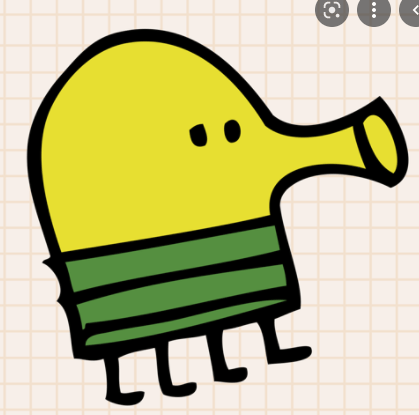← Back to Community

# Binary

Profile icon
JustineHoresh

Hello folks. I need a python program that is converting a number in binary like 2=10.
Thanks in advance for your help and sorry for my broken english

Voters
Profile icon
alpedhuezdewey735
Profile icon
ANGEL6KASNI
Profile icon
JoanDe2
Profile icon
JustineHoresh
hotnewtop
Profile icon
alpedhuezdewey735Profile icon

Decimal To Binary:

print("Converting Decimal Numbers To Binary\n\n")
a=int(input("Enter The Number: "))
b=a%2
print(b)
c=(a//2)%2
print(c)
d=(a//4)%2
print(d)
e=(a//8)%2
print(e)
f=(a//16)%2
print(f)
g=(a//32)%2
print(g)
h=(a//64)%2
print(h)
i=(a//128)%2
print(i)
print("The binary number of the decimal number", a, "is\n", i,h,g,f,e,d,c,b)

Binary To Decimal:

a=str(input("Enter The Number: "))
b=(a[7:8])
c=(a[6:7])
d=(a[5:6])
e=(a[4:5])
f=(a[3:4])
g=(a[2:3])
h=(a[1:2])
i=(a[0:1])
j=int(b)
k=int(c)
l=int(d)
m=int(e)
n=int(f)
o=int(g)
p=int(h)
q=int(i)
print("The Decimal Number Equal To The Entered Binary Number Is", (j2**0)+(k21)+(l*22)+(m2**3)+(n24)+(o*25)+(p2**6)+(q2**7), "\b.")

Completely Working Code...

Profile icon
finleyparr

num = int(input('Input number between 0 and 255 and I will convert it into binary.'))
if num < 256:
print(bin(num))

this code it quite short but it has an annoying 0b at the start and i dont know how to remove it, but as long as you can ignore it or can remove it yourself, feel free to use it, it took me a couple minutes to make

Profile icon
PabloJuan

@finleyparr

bin(int(x)).split('0b')

Profile icon

@finleyparr
Use StrSlicing Code

First Take The Length By Len(#The Number or Variable Here.)
Then Take #The Variable or Number[3:#The Length]

Profile icon
olafh10

I created some code for you: https://repl.it/@olafh10/int-to-binary

This converts the input to binary, note that the program doesn't check if the input is a number. You can also just copy the intToBinary function for in your own program.

Profile icon
JustineHoresh

@olafh10
Thanks you very much

Profile icon
olafh10

@JustineHoresh
No problem!